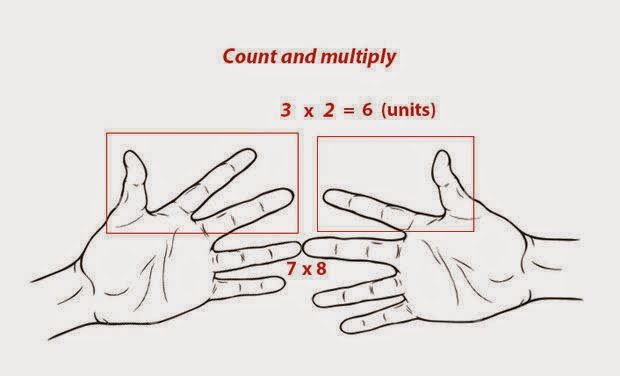If You Learned These Simple Tricks, You'd Never Be Bad At Math --># If You Learned These Simple Tricks, You'd Never Be Bad At MathNowadays, math skills are on a steady decline because almost everyone has a calculator on their phone. But there was a time when we didn't all have calculators in our pockets, when math was actually something you had to learn.

See below a list of 9 simple tricks you can use to figure out complex math problems without a calculator. These tricks will definitely amaze or even shock your friends!### 2. How to Figure Out Multiples of 9### 3. Multiples Of 9 Work In Reverse Order### 4. How To Add and Subtract Fractions### 5. How to Multiply by 11### 6. Figuring Percentages### 7. How to Find Fractions of Whole Numbers### 8. Convert Fahrenheit to Celsius### 9. And The Most Important Tip (!)Category:This site's content is licensed under a Creative Commons Attribution License | Terms of Service | Contact Us